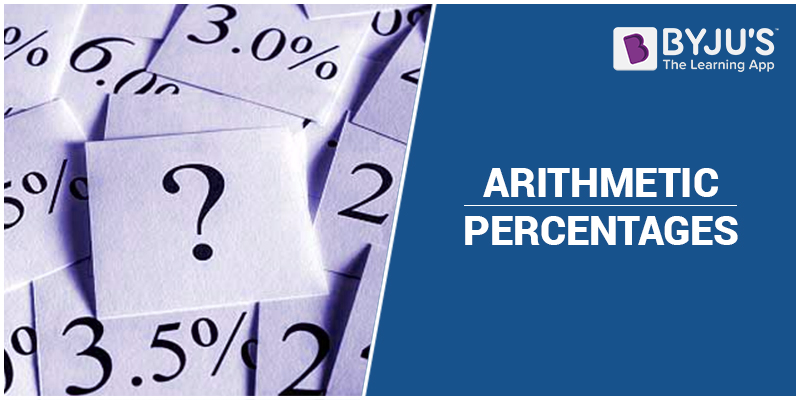# GMAT Quant: Arithmetic – PercentagesPercentage increment is defined as –

$\small \frac{Final \; Value – Initial \; Value}{Initial \; Value} \times 100$

Here we will look at some of the problems which will be asked directly or indirectly in the GMAT exam.

1. In a shop, there were a total of 250 apples and 400 oranges at the beginning of the day. Details of the sales were –

At mid day, 40% of the apples and 20% of the oranges were sold. At the end of   the day, 20% of the remaining apples and 10% of the remaining oranges were sold. Find the total number of fruits sold.

In this problem, typical method will be finding the percentage, subtracting it and again repeating the procedure. We will look at the shortcut or other way of comprehending the problem.

So initially 40% of the apple were sold. That means 60% is left and then 20% is sold hence, remaining is 80%. Therefore, total left apple at the end of the day is $250 \times 0.6 \times 0.8$. Therefore sold apple is

$= 250 – (250 \times 0.6 \times 0.8)$

$= 250 – (1 – 0.6 \times 0.8)$

$= 250 – (1 – 0.48)$

$= 250 \times 0.52$

Similarly sold oranges is

$= 400 (1 – 0.8 \times 0.9)$

$= 400 (1 – 0.72)$

$= 400 \times 0.28$

$= 112$

Therefore total number of fruits sold is 130 + 112 = 242

1. If the price of sugar increase by 20% ,by what percent the family should decrease so the their expenses related to sugar remains constant?

Let the price of 100kg sugar be 100 rupees. New price for 10kg sugar will be $= 100 \times 1.2 = 120$ rupees.

Therefore 100 rupees will be the price of $100 \times \frac{100}{120} = 83.33$. Therefore the reduction will be 100 – 83.33 = 16.66%.

To play with the percentage, you need to know how to play with number 100 and how to convert any fraction to 100. It will be a piece of cake then.

BYJU’S will be glad to help you in your GMAT preparation journey. You can ask for any assistance related to GMAT and MBA from us by calling us at +918884544444. You can write to us at gmat@byjus.com.